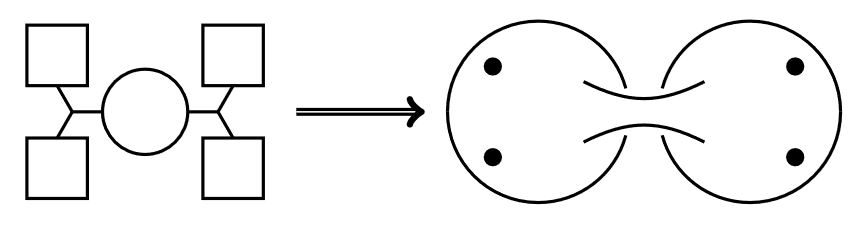nLab AGT correspondence

Context

Duality in string theory

duality in string theory

general mechanisms

string-fivebrane duality

string-string dualities

M-theory

F-theory

string-QFT duality

QFT-QFT duality:

Functorial Quantum field theory

functorial quantum field theory

Contents

Idea

The AGT correspondence (AGT 09) is a relation between

1. the instanton-partition function of $SU(2)^{n+3g-3}$-N=2 D=4 super Yang-Mills theory (Nekrasov’s partition function, e.g. Szabo 15 (2.1))

2. the conformal blocks of Liouville theory on an $n$-punctured Riemann surface $C_{g,n}$ of genus $g$

Here the idea is that $C_{g,n}$ is the super Yang-Mills theory obtained by compactifying the worldvolume 6d (2,0)-supersymmetric QFT of two M5-branes, see at N=2 D=4 super Yang-Mills theory, the section Construction by compactification).

In particular, the N=2 D=4 super Yang-Mills theory is a quiver gauge theory and the correspondence matches the shape of its quiver-diagram to the genus and punctures of the Riemann surface:More generally, this construction yields something like a decomposition of the 6d (2,0)-superconformal QFT into a 2d SCFT “with values in 4d SYM field theory” (e.g. Tachikawa 10, slide 25 (33 of 54)). Hence composition with any kind of suitable invariant of the 4d field theories yields an actual 2d SCFT, for instance taking the superconformal index in 4d yields a 2d TQFT (GPRR 10). In this picture of “4d-SYM field theory-valued 2d SCFT” one has the following correspondences:

Wrapping the M5-brane on a 3-manifold instead yields: 3d-3d correspondence.

A 2d SCFT argued to describe the KK-compactification of the M5-brane on a 4-manifold (specifically: a complex surface) originates with

The origin of the AGT correspondence is:

The 2d TQFT obtained from this by forming the 4d index is discussed in

Relation of the AGT-correspondence to the D=6 N=(2,0) SCFT

and to the 3d-3d correspondence:

Brief surveys include

• Yuji Tachikawa, M5-branes, 4d gauge theory and 2d CFT, 2010 (pdf)

• Abhijit Gadde, $\mathcal{N}= 2$ Dualities and 2d TQFT 2012 (pdf)

• Nikolay Bovev, New SCFTs from wrapped branes, 2013 (pdf)

• Giulio Bonelli, Variations on AGT Correspondence, 2013 (pdf)

• Masato Taki, String Theory as an Attempt of PolyMathematics, talk at 2016.4/28 iTHES-AIMR-IIS (pdf)

More detailed review is in

• Rober Rodger, A pedagogical introduction to the AGT conjecture, Master Thesis Utrecht (2013) (pdf)

• Richard Szabo, $N=2$ gauge theories, instanton moduli spaces and geometric representation theory, Journal of Geometry and Physics Volume 109, November 2016, Pages 83-121 (arXiv:1507.00685)

• Bruno Le Floch, A slow review of the AGT correspondence (arXiv:2006.14025)

• Alexander Belavin, M. A. Bershtein, B. L. Feigin, A. V. Litvinov, G. M. Tarnopolsky, Instanton moduli spaces and bases in coset conformal field theory (arxiv/1111.2803)

• Volker Schomerus, Paulina Suchanek, Liouville’s imaginary shadow (arxiv/1210.1856)

• A.Mironov, A.Morozov, The power of Nekrasov functions (arxiv/0908.2190)

• D. Galakhov, A. Mironov, A. Morozov, S-duality as a beta-deformed Fourier transform (arxiv/1205.4998)

• A. Mironov, Spectral duality in integrable systems from AGT conjecture (arxiv/1204.0913)

• A. Belavin, V. Belavin, AGT conjecture and integrable structure of conformal field theory for $c=1$, Nucl.Phys.B850:199-213 (2011) (arxiv/1102.0343)

• A. Belavin, V. Belavin, M. Bershtein, Instantons and 2d Superconformal field theory (arxiv/1106.4001)

• Kazunobu Maruyoshi, Quantum integrable systems, matrix models, and AGT correspondence, seminar (slides pdf)

• Giulio Bonelli, Alessandro Tanzini, Hitchin systems, N=2 gauge theories and W-gravity (arxiv/0909.4031)

• Giulio Bonelli, Kazunobu Maruyoshi, Alessandro Tanzini, Quantum Hitchin systems via beta-deformed matrix models (arxiv/1104.4016)

• Oscar Chacaltana, Jacques Distler, Tinkertoys for Gaiotto Duality, JHEP 1011:099,2010, (arXiv:1008.5203)

• Satoshi Nawata, Givental J-functions, Quantum integrable systems, AGT relation with surface operator (arXiv/1408.4132)

The AGT correspondence is treated with the help of a Riemann-Hilbert problem in

• G. Vartanov, Jörg Teschner, Supersymmetric gauge theories, quantization of moduli spaces of flat connections, and conformal field theory (arxiv/1302.3778)
category: physics